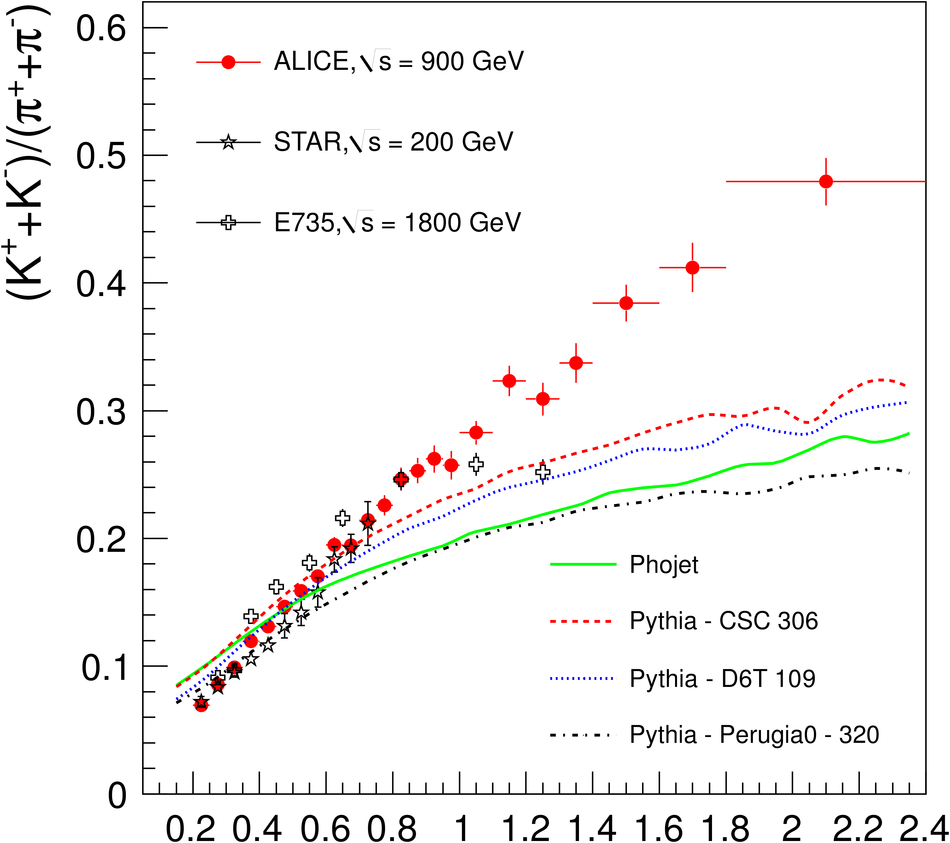# Figure 18

 Ratios of ($K^+$ + $K^-$) / ($\pi^+$ + $\pi^-$)(upper panel) and (p + $\rm\overline{p}$) / ($\pi^+$ + $\pi^-$) (lower panel) as a function of $\pt$ from pp collisions at $\sqrt{s}$ = 900 GeV (statistical errors only). Values from the E735Collaboration  and the STAR Collaboration  (upper part) and from the PHENIX Collaboration  (lower part) also are given. The dashed and dotted curves refer to calculations using PYTHIA and PHOJET at $\sqrt{s}$ = 900 GeV.